Saturday, July 11, 2020
Home > CBSE Class 12 > NCERT Solutions for Class 12 Maths Vector Algebra Exercise 10.4

# NCERT Solutions for Class 12 Maths Vector Algebra Exercise 10.4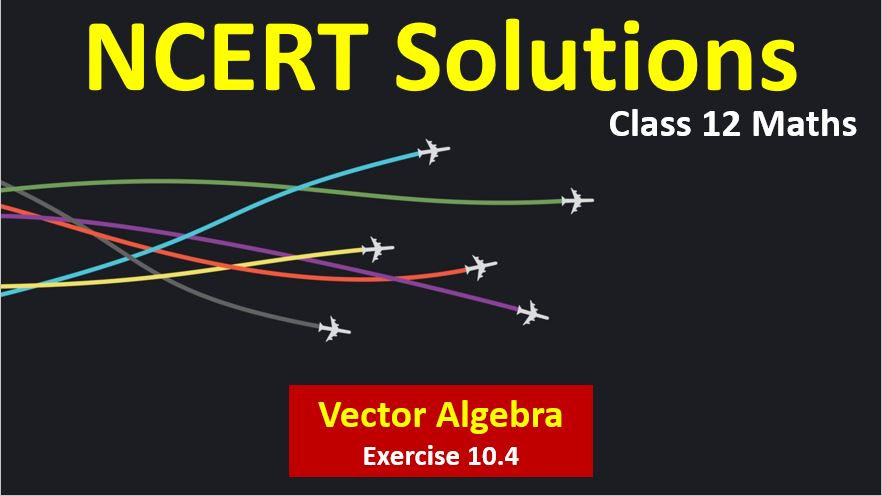# NCERT Solutions for Class 12 Maths Vector Algebra

Hi Students, Welcome to Amans Maths Blogs (AMB). In this post, you will get the NCERT Solutions for Class 12 Maths Vector Algebra Exercise 10.4.

As we know that all the schools affiliated from CBSE follow the NCERT books for all subjects. You can check the CBSE NCERT Syllabus. Thus, NCERT Solutions helps the students to solve the exercise questions as given in NCERT Books.

NCERT Solutions for class 12 is highly recommended by the experienced teacher for students who are going to appear in CBSE Class 12 and JEE Mains and Advanced and NEET level exams. Here You will get NCERT Solutions for Class 12 Maths Vector Algebra Exercise 10.4 of all questions given in NCERT textbooks of class 12 in details with step by step process.

The PDF books of NCERT Solutions for Class 12 are the first step towards the learning and understanding the each sections of Maths, Physics, Chemistry, Biology as it all help in engineering medical entrance exams. To solve it, you just need to click on download links of NCERT solutions for class 12.

CBSE Class 12th is an important school class in your life as you take some serious decision about your career. And out of all subjects, Maths is an important and core subjects. So CBSE NCERT Solutions for Class 12th Maths is major role in your exam preparation as it has detailed chapter wise solutions for all exercise. This NCERT Solutions can be downloaded in PDF file. The downloading link is given at last.

NCERT Solutions for Class 12 Maths are not only the solutions of Maths exercise but it builds your foundation of other important subjects. Getting knowledge of depth concept of CBSE Class 12th Maths like Algebra, Calculus, Trigonometry, Coordinate Geometry help you to understand the concept of Physics and Physical Chemistry.

Note: In this solution, the vector is represented by BOLD font. For example: a, b, OP, AB, i, j, k represent the vectors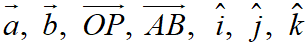## NCERT Solutions for Class 12 Maths Vector Algebra

NCERT Solutions for Class 12 Maths Vector Algebra Exercise 10.4: Ques No 1.

Find | a × b |, if a = i − 7j + 7k and b = 3i − 2j + 2k.

NCERT Solutions:

Given that a = i − 7j + 7k and b = 3i − 2j + 2k.NCERT Solutions for Class 12 Maths Vector Algebra Exercise 10.4: Ques No 2.

Find a unit vector perpendicular to each of the vector (a + b) and (a − b), where a = 3i + 2j + 2k and b = i + 2j − 2k.

NCERT Solutions:

Given that a = 3i + 2j + 2k and b = i + 2j − 2k.

Thus, (a + b) = (3i + 2j + 2k) + (i + 2j − 2k) = (4i + 4j) and

(ab) = (3i + 2j + 2k) − (i + 2j − 2k) = (i + 4k)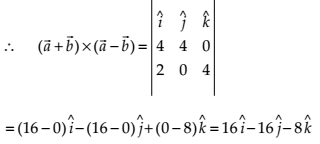Thus, the unit vectors perpendicular to both the vectors (a + b) and (a + b) is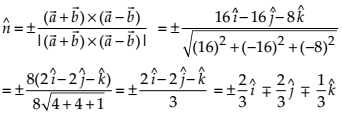NCERT Solutions for Class 12 Maths Vector Algebra Exercise 10.4: Ques No 3.

If a unit vector a makes angles π/3 with i, π/4  with j and an acute angle θ with k, then find θ and hence components of a.

NCERT Solutions: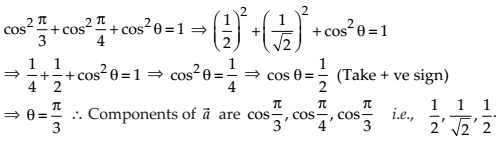NCERT Solutions for Class 12 Maths Vector Algebra Exercise 10.4: Ques No 4.

Show that (ab) × (a + b) = 2(a × b).

NCERT Solutions:

LHS = (ab) × (a + b)

= (a × a) + (a × b) − (b × a) − (b × b

= (0) + (a × b) − [−(a × b)] − (0)     {Since (a × a) = (b × b) = 0 and (b × a) = −(a × b)}

= (a × b) + (a × b) = RHS

NCERT Solutions for Class 12 Maths Vector Algebra Exercise 10.4: Ques No 5.

Find λ and μ if (2i + 6j + 27k) × (i + λj + μk) = 0.

NCERT Solutions:

Given that a = (2i + 6j + 27k) and b = (i + λj + μk).NCERT Solutions for Class 12 Maths Vector Algebra Exercise 10.4: Ques No 6.

Given that a b = 0 and a × b = 0. What can you conclude about the vectors a and b?

NCERT Solutions:

Given that a b = 0 and a × b = 0

a = 0 or b = 0 or ab

and

a = 0 or b = 0 or a || b

⇒ Either a = 0 or b = 0      (Since ab and ab are not true at same time.)

NCERT Solutions for Class 12 Maths Vector Algebra Exercise 10.4: Ques No 7.

Let the vectors a, b, c given as a1i + a2j + a3k, b1i + b2j + b3k, and c1i + c2j + c3k, Then show that a × (b + c) = (a × b) + (a × c).

NCERT Solutions:NCERT Solutions for Class 12 Maths Vector Algebra Exercise 10.4: Ques No 8.

If either a = 0 or b = 0, then a × b = 0. Is the converse true? Justify your answer with an example.

NCERT Solutions:NCERT Solutions for Class 12 Maths Vector Algebra Exercise 10.4: Ques No 9.

Find the area of the triangle with vertices A(1, 1, 2), B(2, 3, 5) and C(1, 5, 5).

NCERT Solutions:NCERT Solutions for Class 12 Maths Vector Algebra Exercise 10.4: Ques No 10.

Find the area of the parallelogram whose adjacent sides are determined by the vectors a = ij + 3k and b = 2i − 7j + k.

NCERT Solutions:NCERT Solutions for Class 12 Maths Vector Algebra Exercise 10.4: Ques No 11.

Let the vectors a and b be such that |a| = 3 and |b| = √2/3, then a × b is a unit vector, if the angle between a and b is

(A) π/6

(B) π/4

(C) π/3

(D) π/2

NCERT Solutions:

(B) Since |a × b| = |a||b|sinθNCERT Solutions for Class 12 Maths Vector Algebra Exercise 10.4: Ques No 12.

Area of a rectangle having vertices A, B, C and D with position vectors, respectively is

(A) 1/2

(B) 1

(C) 2

(D) 4

NCERT Solutions:

(C)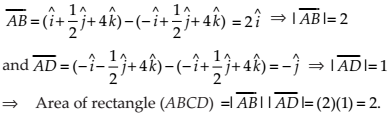error: Content is protected !!